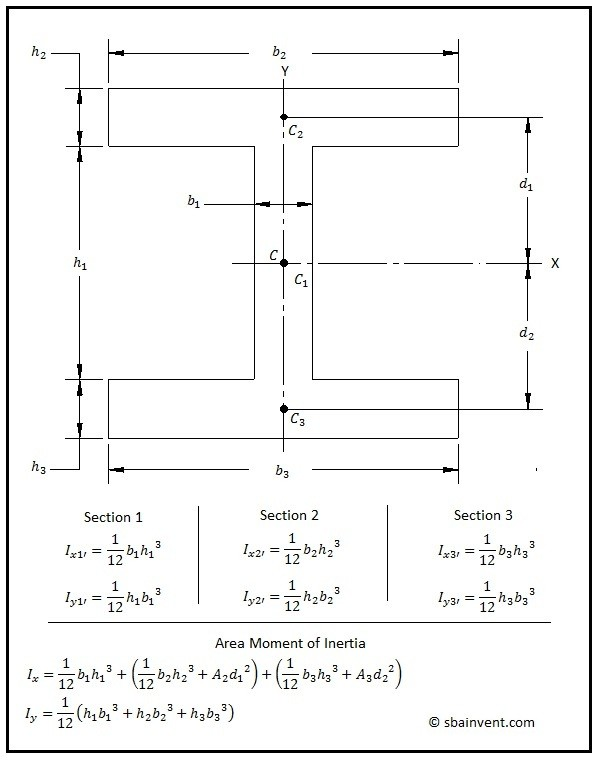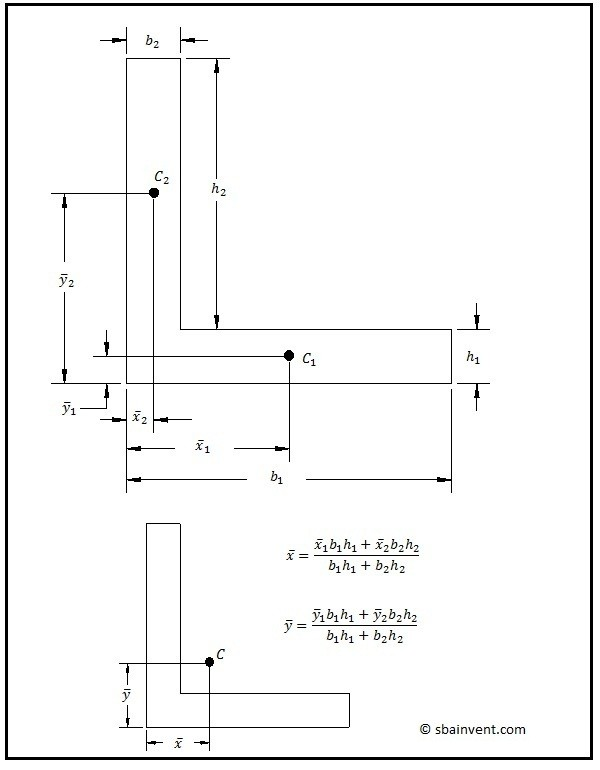# Area Moment of Inertia

Knowing the area moment of inertia is a critical part of being able to calculate stress on a beam. It is determined from the cross-sectional area of the beam and the central axis for the direction of interest. For basic shapes there are tables that contain area moment of inertia equations which can be viewed below. However, there are certain cases where the area moment of inertia will have to be calculated either through calculus or by manipulating the equations found in this table.##### Area Moment if Inertia Calculator
 Width (b): Height (h): Radius (r): Rectangle Triangle Semicircle Circle Answer =

To calculate the area moment of inertia through calculus equation 1 would be used for a general form.

(Eq 1)  $I_{xy}=\int{xy}da$

##### Multiple Sectioned Beams

As mentioned earlier in some cases, such as an I-beam, the equations above would have to be manipulated to calculate the area moment of inertia for that shape. To calculate the area moment of inertia equations 2 and 3 would be used. Refer to the example below for a better understanding.

(Eq 2)  $I_x=\sum{(I_{x’}+Ad_y^2)}$

$I_{x’}$ = Area Moment of Inertia of Each Segment

$A$ = Area of Each Segment

$d_y$ = Distance from Segment Centroid to Beam’s Centroid

(Eq 3)  $I_y=\sum{(I_{y’}+Ad_x^2)}$##### Finding the Centroid of a Beam

On the figures above you may have noticed the letter C next to a dot. This is the centroid of the part. The centroid is important in determining the area moment of inertia because, as seen in the previous example, sections relate of the centroid. Basically what the centroid does is it splits the area of the cross-section evenly across an x and y axis. To determine the centroid, equations 4 and 5 would be used, or you could take symmetry into consideration if it applies. Refer to the example below.

(Eq 4) $\bar{x}=\frac{\sum{\bar{x’}}} {\sum{A}}$

$\bar{x}’$ = Distance to Centroid of Sections

(Eq 5) $\bar{y}=\frac{\sum{\bar{y’}}} {\sum{A}}$